Reach Us+44-1625-708989
Comparison of Macrodosimetric Efficacy of Transarterial Radioembolization (TARE) by Using 90Y Microspheres of Different Density of Activity | OMICS International
Journal of Physical Mathematics
All submissions of the EM system will be redirected to Online Manuscript Submission System. Authors are requested to submit articles directly to Online Manuscript Submission System of respective journal.

# Comparison of Macrodosimetric Efficacy of Transarterial Radioembolization (TARE) by Using 90Y Microspheres of Different Density of Activity

Traino AC1*, Piccinno M1, Boni G2, Bargellini I3 and Bozzi E3

1Unit of Medical Physics, University Hospital Pisana, Italy

2Unit of Nuclear Medicine, University Hospital Pisana, Italy

3S.D Radiologia Vascular and Interventional, University Hospital Pisana, Italy

*Corresponding Author:
Traino AC
Professor, Unit of Medical Physics
University Hospital Pisana, Italy
Tel: +39 050992957
E-mail: [email protected]

Received Date: July 01, 2015; Accepted Date: January 11, 2016; Published Date: January 15, 2016

Citation: Traino AC, Piccinno M, Boni G, Bargellini I, Bozzi E (2016) Comparison of Macrodosimetric Efficacy of Transarterial Radioembolization (TARE) by Using 90Y Microspheres of Different Density of Activity. J Phys Math 7:150. doi:10.4172/2090-0902.1000150

Copyright: © 2016 Traino AC, et al. This is an open-access article distributed under the terms of the Creative Commons Attribution License, which permits unrestricted use, distribution, and reproduction in any medium, provided the original author and source are credited.

Visit for more related articles at Journal of Physical Mathematics

#### Abstract

Purpose: Transarterial 90Y microspheres radioembolization is emerging as a multidisciplinary promising therapeutic modality for primary and metastatic cancer in the liver. Actually two different type of microspheres are used, whose main characteristic is the different density of activity (activity per microsphere). In this paper the effect due to the possible different distribution of the microspheres in a target is presented and discussed from a macrodosimetric point of view. Material and methods: A 100 g virtual soft-tissue target region has been builded. The administered activity was chosen to have a target average absorbed dose of 100 Gy and the number of 90Y microspheres needed was calculated for two different activity-per-microsphere values (2500) Bq/microsphere and 50 Bq/microsphere, respectively). The spheres were randomly distributed in the target and the Dose Volume Histograms were obtained for both. The cells surviving fractions (SF) for four different values of the radiobiological parameter α were calculated from the Linear - Quadratic model. Results: The DVH obtained are very similar and the SF is almost equal for both the activity-per- microsphere values. Conclusions: This macrodosimetric approach shows no radiobiological difference between the glass and resin microspheres. Thus the different number of microspheres seems to have no effect when the number of spheres is big enough that the distance between the spheres in the target can be considered small compared to the range of theÃ¯Â€Â  β-particles of 90Y.

#### Introduction

Radioembolization with 90y microspheres via hepatic arterial administration has been shown to be effective in the treatment of primary and metastatic liver cancer (HCC), as well as in unresectable colon carcinoma metastases [1-5] Radioembolization is a locoregional liver directed therapy that involves transcatheter delivery of microspheres embedded with 90y. 90y microspheres are injected into the arterial supply of the liver, where they preferentially flow into hyper vascularized tumor zones, with a higher irradiation of tumour tissue compared to the normal liver parenchyma, with a consequent tumourtissue necrosis. Actually 90y can be delivered to the hepatic tumor as either a constituent of a glass microsphere, TheraSphere®, or as a biocompatible resin-based microsphere, SIR-Spheres®. TheraSphere® was approved by the USA Food and Drug Administration for unresectable HCC in December 1999 under a Humanitarian Device Exemption. SIR-Spheres® was approved in March 2002 for colorectal cancer metastatic to the liver in conjunction with continuous infusion of intrahepatic floxuridine (FUDR) .

The characteristics of these two different kinds of90Y microspheres are summarized in Table 1 . From a dosimetric point of view, the main difference between SIR-Spheres® and TheraSphere® is the density of activity that in one case (SIR-Spheres® whose activity per microsphere is ~50 Bq) is much lower than in the other (TheraSphere® whose activity per microsphere is 2500 Bq).

90Y-Microspheres
Material Resin Glass
Sphere size (mm) 20-60 20-30
Activity per sphere (Bq) 40-70 2500
Specific gravity Low High
Handling for dispensing Required Not required
Splitting one vial for more patients Possible Not possible

Table 1: Main characteristics of the two available kinds of 90Y microsphere: Glass spheres (TheraSphere®) and resin spheres (SIR-Spheres®).

In principle this difference could have an impact on the radiobiological effectiveness of the treatment , due to the much higher number of microspheres needed to have the same activity in the target tissue in one case (SIR-Spheres®) compared to the other (TheraSphere®). This because an higher number of microspheres could mean a more homogeneous distribution of activity (and consequently of target absorbed dose).

In this paper a comparison of these two different densities of activity is presented and discussed, showing that, neglecting other differences between the two types of90Y microspheres (different material and consequent different specific gravity of SIR-Spheres® compared to TheraSphere®, for example) the therapeutic effectiveness of the two radioembolization tools is almost the same from a macrodosimetric point of view.

#### Materials and Methods

A cubical target was simulated to test the expected difference between the two different activities-per- sphere tools. The mass of the target was 100 g, its density was 1.04 g/cm3, that is the density of the soft tissues . The activity needed for an average target absorbed dose of 100 Gy was calculated by using the MIRD formalism: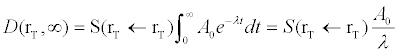where D(rT,∞)=100 Gy is the target absorbed dose; S(rT,rT )=5.08 mGy/ MBq*h is the S-value for a self-irradiating 100 g spherical target treated with90Y; A0=administered activity and λ=0.011 h-1 is the physical decay constant of 90Y. From Equation 1 it follows A0=213 MBq.

The target volume was divided into N=21x21x21 square voxels of 2.21 mm size.

A number nsph of90Y embedded microspheres of the same size were randomly distributed into the target, according with the equation: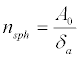Where δa represents two different densities of activity of 2500 Bq/ sphere and 50 Bq/sphere respectively. For an administered activity of 213 MBq there will be nsph =8.52 × 104 90Y microspheres corresponding to a density of activity δa =2500 Bq/sphere (glass spheres) and nsph =4.26 × 106 90Y microspheres corresponding to δa =50 Bq/sphere (resin spheres). The size was considered the same, 30μm, for both the type of microspheres.

Software was built by using the open-source environment GNUOctave to randomly distribute a number nsph of microspheres in the target, to perform a 3D dosimetric calculation at the voxel level by using the MIRD 17 method  and to show the Dose Volume Histogram (DVH) corresponding to the different distributions of the 90Y microspheres.

The absorbed dose di in each voxel was calculated, according to the MIRD 17 method, by using the equation: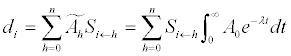where Ãh is the cumulated activity in the ith-voxel and Si←h are the dose conversion factors at the voxel level. The Si←h values for the voxel size of 2.21 mm were taken  Note that Ah is the activity in each h voxel, calculated by multiplying the number of microspheres randomly placed in that voxel by the density of activity δa.

#### Results

The Dose Volume Histograms for δa =2500 Bq/sphere and δa =50 Bq/sphere are shown in Figure 1. DVHs for the two values of δa considered are very similar.

Figure 1: Integral DVH in a target region of 100 g calculated for δa=2500 Bq/ sphere and δa=50Bq/sphere respectively. The administered activity A0=213 MBq, needed to have an average absorbed dose of 100 Gy from the target region.

The surviving fraction SF was calculated for each of the two values of δa by using the Linear-Quadratic (LQ) model: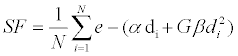In Equation 4 β=/10 and the Lea-Catcheside factor G=0.023. This last factor for a mono-exponential decreasing dose-rate can be written as .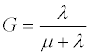Where μ=ln(2)/ trep is the rate of repair of sub-lethal damages (the repair half-time constant trep=1.5 h for tumor lesions was extracted from Strigari  and λ=0.011h-1 is the physics decay rate of 90Y. The cells surviving fraction for four different values of α (α =1; 0.1; 0.01 and 0.001 Gy-1) is shown in Table 2.

Surviving  Fraction
a (1/Gy) 2500 Bq/sphere 50 Bq/sphere
1 5.90E-21 4.30E-27
0.1 1.02E-04 6.00E-05
0.01 0.32 0.32
0.001 0.89 0.89

Table 2: Cells surviving fractions for different values of a and different values of δ a (2500 Bq/sphere and 50 Bq/sphere). The calculation was based on equation 4 with a /b=10 and G=0.023.

#### Discussion and Conclusions

From a macrodosimetric point of view, the different number of 90Y microspheres per unit mass could have an impact on the radiobiological effect of the transarterial radioembolization therapy , due to the different distribution of the microspheres in the treated target region. The microspheres tend to be distributed as homogeneously as possible via the microvascularization of the target zone. The homogeneous distribution of the activity represents the better situation from a radiobiological point of view, because it causes an uniform absorbed dose by the target. In this paper the hypothesis of a random distribution of the microspheres in the target has been done, without considering the trapping of the spheres in the blood vessels.

If the microspheres are rarefied in the target, meaning that the average distance between spheres is higher than than the double range of the β- of the radionuclide employed (90Y in this case), the dose distribution will be very unhomogeneous, leaving target zones where the absorbed dose is zero Figure 2.

Figure 2: Representation of a rarefied distribution of microspheres in the target. If the distance between two microspheres is bigger than the double range of the β-particles there is a no-absorbed energy (and then noabsorbed dose) zone. This means a very unhomogeneous absorbed dose distribution in the target.

In the case described in this paper, doing the hypothesis of a random distribution of the 90Y microspheres in the target, for a 100 g target treated with an activity of 213 MBq, which corresponds to a target absorbed dose of 100 Gy, the average density of 90Y microspheres is 0.852 spheres/mm3 if δa =2500 Bq/sphere and 42.6 spheres/mm3 if δa =50 Bq/sphere. Remembering that the range of the β- particles of 90Y is about 11 mm in the soft tissues, this means that the β- Particles of the 90Y are close also for δa =2500 Bq/sphere, compared to their range. Thus for the glass microspheres (δa =2500 Bq/sphere) there are low high-activity spheres for unit mass, compared to an higher number of low-activity resin microspheres (δa =50 Bq/sphere). In both cases the effect of the microspheres is almost homogeneous in the volume considered, because the average distance among the spheres is lower than the range of the β- particles of 90Y Figure 3.

Figure 3: 2D representation of the target region, divided into cubical voxels of 2.21 mm size. The 90Y microspheres are randomly distributed in the voxels. The average number of glass microspheres per voxel is 9.2; the average number of resin microspheres per voxel is 459.8. The microspheres are closer than the β- range of the 90Y (maximum range: 11 mm; average range: 2.5 mm)

Figure 4: Representation of the real distribution of the 90Y microspheres in the target. Themicrospheres are vehiculated in the target by the arterial vascularization, thus their distributioncannot be homogeneous. The dotted lines represent the isodose curves in the target.

In Table 3 the average density (number of spheres/mm3) of 90Y glass and resin microspheres (δa=2500 Bq/sphere and δa =50 Bq/sphere respectively) needed for an average target absorbed dose of 100 Gy is shown for different target mass values. The average number of spheres per mm3 of target has a very low variation depending on target mass. This means that the macrodosimetric effect of the glass and resin 90Y microspheres is almost the same in the target, because the microspheres are very close respect to the range of the β- particles of 90Y. This seems true for all the possible treated masses, if the required target average absorbed dose is 100 Gy.

TARGET MASS (g) AMINISTERED ACTIVITY (MBq) AVERAGE NUMBER OF GLASS SPHERES/mm3 AVERAGE NUMBER OF RESIN SPHERES/mm3
40 86 0.866 43.3
80 170 0.854 42.7
100 213 0.853 42.6
300 626 0.835 41.7
400 833 0.833 41.7
500 1041 0.833 41.7
100 2059 0.825 41.2
2000 4026 0.805 40.3

Table 3: Density (number of spheres/mm3) of glass and resin microspheres (δa =2500 Bq/sphere and δa =50 Bq/sphere respectively) for different masses of softtissue target. The administered activity corresponds to an average target absorbed dose of 100 Gy; the density of the soft-tissue is 1.04 Kg/dm3.

The random distribution of the microspheres in the whole target is only a hypothesis. The real situation is different because the microspheres are vehiculated into the target by the arterial system: this means that the distribution of the spheres in the target is unhomogeneous in principle. In the real situation the 90Y activity needed to have a certain absorbed dose (es.100 Gy) in a target volume (es. 100 g) is forced in a volume smaller than the target (arterial system in the target is smaller than the whole target). This means that there is the same number of microspheres (8.52 × 104 90Y microspheres corresponding to a density of activity δa =2500 Bq/sphere and 4.26x106 corresponding to δa =50 Bq/sphere) randomly distributed in a volume lower than 100 g. For this reason the 90Y microspheres are much closer than the situation described in this paper, thus the macrodosimetric differences due to the different number of resin and glass microspheres in the target are probably lower.

In this paper the macrodosimetric effect due to the different number of 90Y microspheres per unit mass in a target submitted to the radioembolization procedure is treated. From this point of view, it seems that the different number of microspheres doesn't have any effect on the distribution of the target absorbed dose due to the small distance among the spheres compared to the range of the particles of 90Y. This result seems to be in agreement with those described by Gulec in their paper , where, starting from a microdosimetric approach, they don't find any difference in the absorbed dose due to the different number of microspheres in the target.

The microdosimetic effects due to the different density of activity (activity per sphere) between glass and resin microspheres, already treated in literature [13,14] is beyond the scope of this paper. Also the probable radiobiological effect due to the different specific gravity of the microspheres (due to the different material), which almost surely affects the distribution of the microspheres in the target, is not taken into consideration in this paper.

#### References

Select your language of interest to view the total content in your interested language

### Article Usage

• Total views: 8613
• [From(publication date):
March-2016 - Jul 23, 2019]
• Breakdown by view type
• HTML page views : 8431Can't read the image? click here to refresh